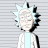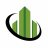# Finite Volume Elements (FVE) for ThinkOrSwim#### Pelonsax

##### Active member
VIP
Would you mind sharing the study code or chart with the fix, please?

Code:
``````#
# FiniteVolumeElements_FVE
#
# Author: Kory Gill, [USER=212]@korygill[/USER]
#
# Created from idea on https://usethinkscript.com/threads/finite-volume-elements.593/
# Original idea is from http://mkatsanos.com/FVE.html
#
# VERSION HISTORY (sortable date and time (your local time is fine), and your initials
# 20190907-1200-KG    - Created.
# ...7/20/2020 Added MTF Capability in order to combat the fact that it does not appear on lower than 2 minute time frames### Ramon Del Villar AKA Pelonsax
# ...

declare lower;
input AGG = aggregationPeriod.FIVE_MIN;
input CutOff = 0.003;
input Samples_Used = 22;
def samples = Samples_Used * (AGG/60000);

def bn = BarNumber();
def nan = double.NaN;

def tp = hlc3(period = AGG);
def mf = (close(period = AGG) - (high(period = AGG)+low(period = AGG))/2) + tp - tp;
def fveFactor = if mf > (CutOff*Close(period = AGG))
then 1
else if mf < (-1*CutOff*Close(period = AGG)) then -1
else 0;

def VolumePlusMinus = volume(period = AGG) * fveFactor;
def fveSum = Sum(VolumePlusMinus, Samples);
def fve = if bn > Samples
then (fveSum / (Average(volume(period = AGG),Samples) * Samples)) * 100
else nan;

plot pFVE = fve;

plot pFVE_MA = MovingAverage(AverageType.SIMPLE, fve, 30);
pFVE_MA.SetDefaultColor(Color.Gray);
PFVE_MA.SetPaintingStrategy(PaintingStrategy.DASHES);

plot zeroLine = 0;
zeroLine.SetDefaultColor(Color.White);``````

•korygill#### Pelonsax

##### Active member
VIP
I'VE DONE IT!!!!!!!! OMFG I CAN'T BELIEVE I DID IT!!! (Okay, truth is, this is a little over my head, but I think I did it. You be the judge)

Spike_Dampened_Finite_Volume_Elements (I suppose another way of calling it could be "NORMALIZED_FINITE_VOLUME_ELEMENTS")

I took the logic from Volume Flow Indicator which is a normalized version of On Balance Volume and applied it here. Below are the indicators in question back to back. Previously, I had thought that the only way to show the original FVE study on a one minute chart was to change the aggregation, but as you can see, there is no need to do that with this "Normalized" Version (though it is possible if one were so inclined.Here is the code to the dampened indicator:

Code:
``````# FiniteVolumeElements_FVE
#
# Author: Kory Gill, [USER=212]@korygill[/USER]
#
# Created from idea on https://usethinkscript.com/threads/finite-volume-elements.593/
# Original idea is from http://mkatsanos.com/FVE.html
#
# VERSION HISTORY (sortable date and time (your local time is fine), and your initials
# 20190907-1200-KG    - Created.

###############################################################################
### NORMALIZATION (Volume Damping adaptation) by Ramon Del Villar AKA PElonsax
### 7/20/2020 #################################################################
###############################################################################
# The damping logic is taken directly from VFI #

declare once_per_bar;
declare lower;

#
# inputs
#
input Samples = 22;

#
# logic
#
def bn = BarNumber();
def nan = double.NaN;

input maxVolumeCutOff = 2.5;
###Coefficient used in calculation of “Cut Off” value. Lower values mean stronger damping of volume spikes###

Assert(maxVolumeCutOff > 0, "'max volume cut off' must be positive: " + maxVolumeCutOff);

def cutOff = 0.2 * StDev(Log(hlc3) - Log(hlc3), 30) * close;
def hlcChange = hlc3 - hlc3;
def avgVolume = Average(volume, 30);
def minVolume = Min(volume, avgVolume * maxVolumeCutOff);
def dirVolume = if hlcChange > cutOff
then minVolume
else if hlcChange < -cutOff
then -minVolume
else 0;

def mf = (close - (high+low)/2) + hlc3 - hlc3 ;
def fveSum = Sum(dirVolume, Samples);

def fve = if bn > Samples
then (fveSum / (Average(avgVolume, Samples) * Samples)) * 100
else nan;

#
# plots
#
plot pFVE = fve;

plot pFVE_MA = MovingAverage(AverageType.SIMPLE, fve, 30);
pFVE_MA.SetDefaultColor(Color.Gray);
PFVE_MA.SetPaintingStrategy(PaintingStrategy.DASHES);

plot zeroLine = 0;
zeroLine.SetDefaultColor(Color.White);``````

https://tos.mx/asxD578
ENJOY!!

••diazlaz, korygill, Piper792 and 2 others
D

#### dinodotcom

##### Member
2019 Donor
This looks fascinating. I can see how this would be very valuable. Would you mind offering a small bit of commentary on how exactly you use this?

Also would you mind sharing the MTF volume elements code also?

Last edited by a moderator:
•Pelonsax
P

#### petey150

##### New member
@MerryDay Could you add another longer MA to it??#### CoryW

##### New member
VIP
This looks fascinating. I can see how this would be very valuable. Would you mind offering a small bit of commentary on how exactly you use this?

Also would you mind sharing the MTF volume elements code also?
Here you go! https://tos.mx/m9wT4jz#### MerryDay

##### Active member
VIP
@petey150

Code:
``````# to chg the length of the moving average, change line#17: The "30" represents length
def FVE_MA = MovingAverage(AverageType.SIMPLE, fve, 30);``````

•petey150How to scan for call volume greater than a % of outstanding shares Questions 5Volume Oscillator for ThinkorSwim Indicators 2